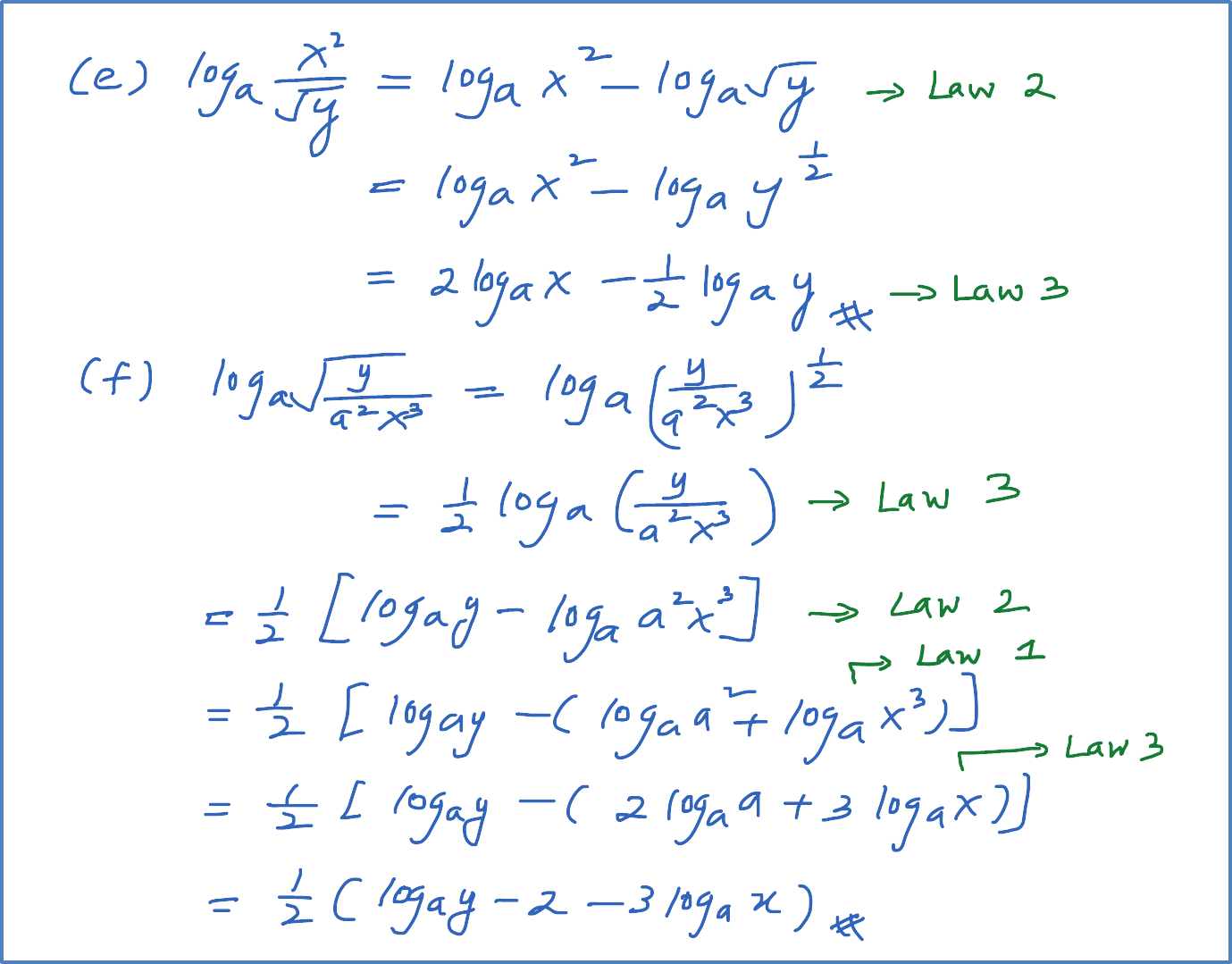\

# 4.2.1 Laws of Logarithms

4.2a Laws of Logarithms

Example 1

Express the following in term of ${\mathrm{log}}_{a}x$   and ${\mathrm{log}}_{a}y$  .
(a) ${\mathrm{log}}_{a}3x$
(b) ${\mathrm{log}}_{a}\frac{x}{5}$
(c) ${\mathrm{log}}_{a}{y}^{5}$
(d) ${\mathrm{log}}_{a}x{y}^{3}$
(e) ${\mathrm{log}}_{a}\frac{{x}^{2}}{\sqrt{y}}$
(f) ${\mathrm{log}}_{a}\sqrt{\frac{y}{{a}^{2}{x}^{3}}}$

Law of Logarithms